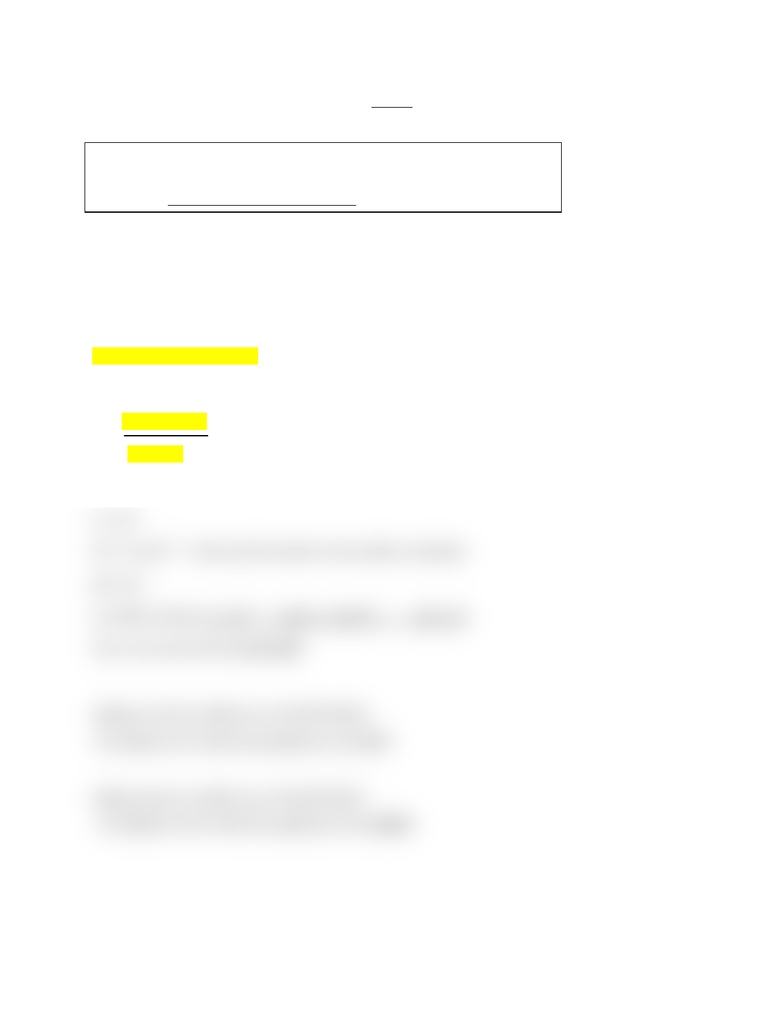Class Notes (1,100,000)
CA (630,000)
Langara (1,000)
CHEM (10)
Lecture

CHEM 1114 Lecture Notes - Conjugate Acid, Acid Dissociation Constant, Buffer Solution

by

Department
Chemistry
Course Code
CHEM 1114
Professor
K.Sveinson

This preview shows half of the first page. to view the full 2 pages of the document.Buffers
Buffer : a solution containing a weak acid and its conjugate base in equilibrium
(or a weak base and its conjugate acid in equilibrium)
Both components of the situation are in appreciable quantities (> 1.0 M)
Note: acid and conjugate bases do not have to have the same concentration, but close
Ex. Consider a 1M CH3COOH buffer solution
CH3COOH  CH3COO- + H+
Ka is equal to products / reactants therefore :
Ka = [CH3COOH] [H+]
CH3COOH
Since CH3COOH = CH3COO , they can cancel out leaving..
Ka = [H+]
[H+] = 1.8 x10 -15 (look up the bronsted lowry table to calculate)
pH= 4.74
In a buffer solution, Ka = [H+] <-log Ka = -log [H+] ; pKa = pH
Thus, we can also infer that pH =pKa
Adding an acid to a buffer (e.g. CH3COOH buffer)
-The addition of H+ shifts the equilibrium to the LEFT
Adding a base to a buffer (e.g. CH3COOH buffer)
- The addition of OH- shifts the equilibrium to the RIGHT
You're Reading a Preview

Unlock to view full version